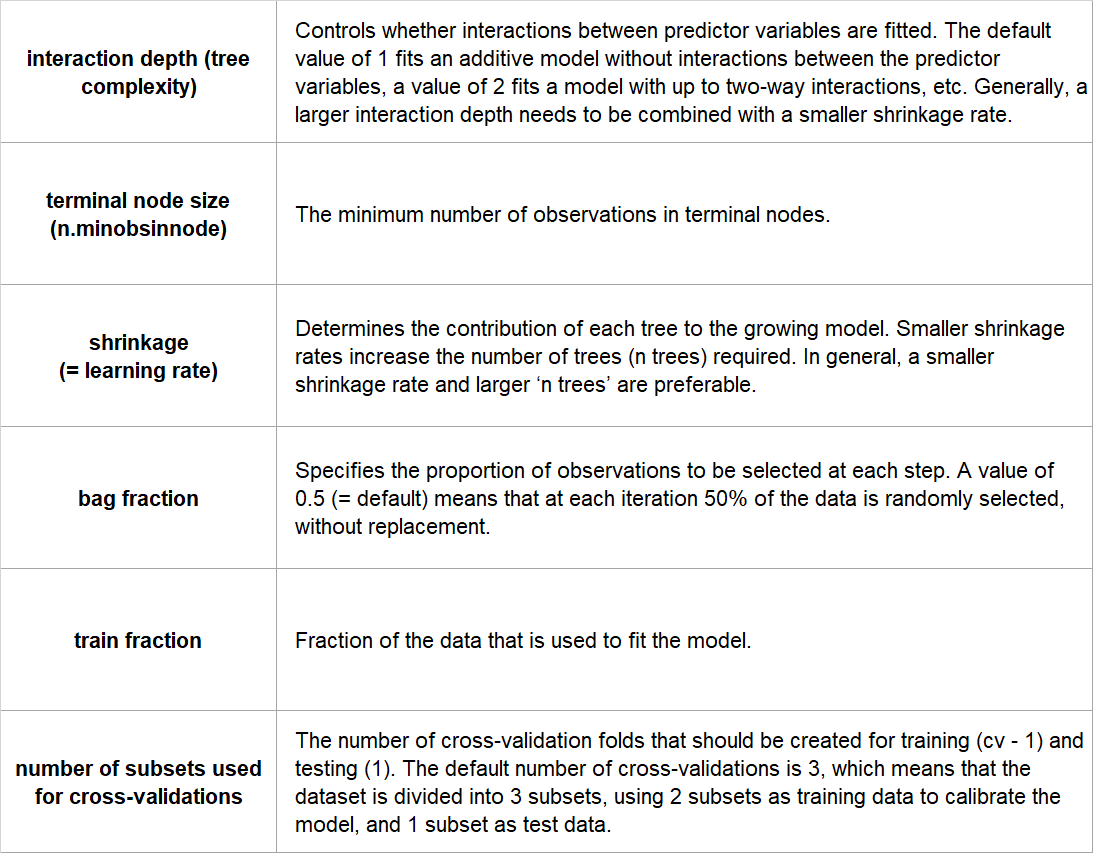## Introduction

This model is similar to Boosted Regression Trees only run through a different package in R.

These models are a combination of two techniques: decision tree algorithms and boosting methods. Generalized Boosting Models repeatedly fit many decision trees to improve the accuracy of the model. For each new tree in the model, a random subset of all the data is selected using the boosting method. For each new tree in the model the input data are weighted in such a way that data that was poorly modelled by previous trees has a higher probability of being selected in the new tree. This means that after the first tree is fitted the model will take into account the error in the prediction of that tree to fit the next tree, and so on. By taking into account the fit of previous trees that are built, the model continuously tries to improve its accuracy. This sequential approach is unique to boosting.

Generalized Boosting Models have two important parameters that need to be specified by the user.

• Interaction depth (= tree complexity in BRT): this controls the number of splits in each tree. A value of 1 results in trees with only 1 split, and means that the model does not take into account interactions between environmental variables. A value of 2 results in two splits, etc.
• Shrinkage (= learning rate in BRT): this determines the contribution of each tree to the growing model. As small shrinkage value results in many trees to be built.

These two parameters together determine the number of trees that is required for optimal prediction. The aim is to find the combination of parameters that results in the minimum error for predictions, and a model with at least 1000 trees. The optimal values for these parameters depend on the size of your dataset. For datasets with <500 occurrence points, it is best to model simple trees (interaction depth = 2 or 3) with small enough shrinkage rates to allow the model to grow at least 1000 trees.

Generalized Boosting Models are a powerful algorithm and work very well with large datasets or when you have a large number of environmental variables compared to the number of observations, and they are very robust to missing values and outliers.

• Can be used with a variety of response types (binomial, gaussian, poisson)

• Stochastic, which improves predictive performance

• The best fit is automatically detected by the algorithm

• Model represents the effect of each predictor after accounting for the effects of other predictors

• Robust to missing values and outliers

## Limitations

• Needs at least 2 predictor variables to run

## Assumptions

No formal distributional assumptions, generalized boosting models are non-parametric and can thus handle skewed and multi-modal data as well as categorical data that are ordinal or non-ordinal.

Yes.

## Configuration options

GBM, which uses the ‘gbm’ package, implemented in biomod2. The user can set the following configuration options:## References

• De'Ath G (2007) Boosted trees for ecological modeling and prediction. Ecology, 88(1): 243-251.
• Elith J, Leathwick JR, Hastie T (2008) A working guide to boosted regression trees. Journal of Animal Ecology, 77(4): 802-813.
• Franklin J (2010) Mapping species distributions: spatial inference and prediction. Cambridge University Press.
• Ridgeway G (1999) The state of boosting. Computing Science and Statistics, 172-181.
• Thuiller W, Lafourcade B, Araujo M (2012) Presentation manual for BIOMOD. Laboratoire d'Écologie Alpine, Université Joseph Fourier, Grenoble, France